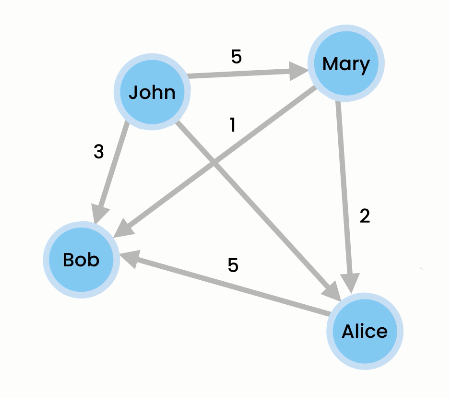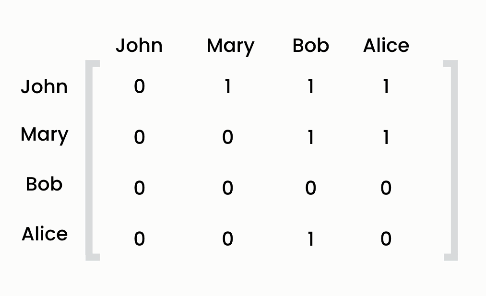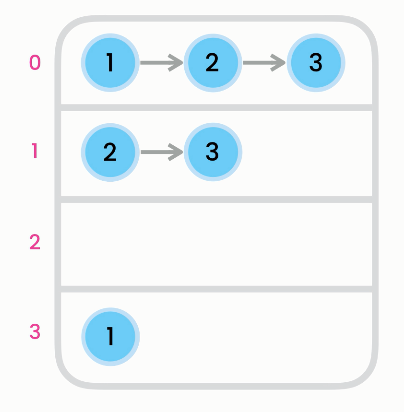Graphs

# Graphs

2020-02-13T23:46:37.003Z

Graphs represent relationships between entities—graphs convey how data is connected. Graphs have no root, only nodes (or vertices) and edges. If two nodes are directly connected, we say they are adjact or neighbors. If the edges have a direction, we say it is a directed graph. Connections may have a weight, i.e. how strong the connection is.GraphDirected and undirected graph

Trees as graphs

A tree is actually a special case of a graph—a tree is simply a graph without a cycle. A tree is a special case of a graph where each node (except the root) has exactly one node referencing it (its parent) and there are no cycles.

Unlike tree nodes, graph nodes (or vertices) can have multiple parents. In addition, the links between nodes may have values or weights. These links are called "edges" because they may contain more information than just a pointer. In a graph, edges can be one-way or two-way. A graph with one-way edges is called a "directed graph". A graph with only two-way edges is called an "undirected graph". Graphs may have cycles, so when these algorithms are applied to graphs, some mechanism is needed to avoid re-traversing parts of the graph that have already been visited.

Graphs can be implemented with an adjacency matrix:When we do time complexity analysis on graphs, we use `V` (for vertices) and `E` (for edges). For example, adding an item to and removing an item from an adjacency matrix is `O(V^2)` because we have to copy all the other items around. Adding and removing an edge as well as querying an edge is `O(1)` because we use a hash table to keep the vertices indexed. Finding the neighbors of a node runs in `O(V)`.Graphs can also be implemented with an adjacency list (an array of linked lists):A dense graph is one where all vertices are connected to all other vertices. If you are dealing with a dense graph, use an adjacency matrix; otherwise, use an adjacency list in most cases.

A graph may be directed or undirected. In a directed graph, each edge has a direction, which indicates that you can move from one vertex to the other through the edge. In an undirected graph, you can move in both directions between vertices.

Two vertices in a graph are said to be adjacent if they are connected by the same edge. Similarly, two edges are said to be adjacent if they are connected to the same vertex. An edge in a graph that joins two vertices is said to be incident to both vertices. The degree of a vertex is the number of edges incident to it.

Two vertices are called neighbors if they are adjacent. Similarly, two edges are called neighbors if they are adjacent.

You can represent a graph using an adjacency matrix or adjacency lists. Which one is better? If the graph is dense (i.e., there are a lot of edges), using an adjacency matrix is preferred. If the graph is very sparse (i.e., very few edges), using adjacency lists is better, because using an adjacency matrix would waste a lot of space.

There are two types of weighted graphs: vertex weighted and edge weighted. In a vertexweighted graph, each vertex is assigned a weight. In an edge-weighted graph, each edge is assigned a weight. Of the two types, edge-weighted graphs have more applications.

## Implementation

``````public class Graph {
private class Node {
private String label;
Node(String label) {
this.label = label;
}

@Override
public void toString() {
return label;
}
}
private Map<String, Node> nodes = new HashMap<>();
private Map<Node, List<Node>> adjacencyList = new HashMap<>();
// this implementation uses maps, but still considered an adjacency list

public void addNode(String label) {
var node = new Node(label);
nodes.putIfAbsent(label, node);
}

public void addEdge(String from, String to) {
var fromNode = nodes.get(from);
if (fromNode == null)
throw new IllegalArgumentException();

var toNode = nodes.get(to);
if (toNode == null)
throw new IllegalArgumentException();

}

public void print() {
for (var source : adjecencyList.keySet()) {
var targets = adjacencyList.get(source)
if (!targets.isEmpty()) {
System.out.println(source + " is connected to " + targets);
}
}
}

public void removeNode(String label) {
var node = nodes.get(label);
if (node == null)
return;

// iterate over every node in this graph
for (var n : adjacencyList.keySet()) {
}
nodes.remove(node);
}

public void removeEdge(String from, String to) {
var fromNode = nodes.get(from);
var toNode = nodes.get(to);

if (fromNode == null || toNode == null)
return;